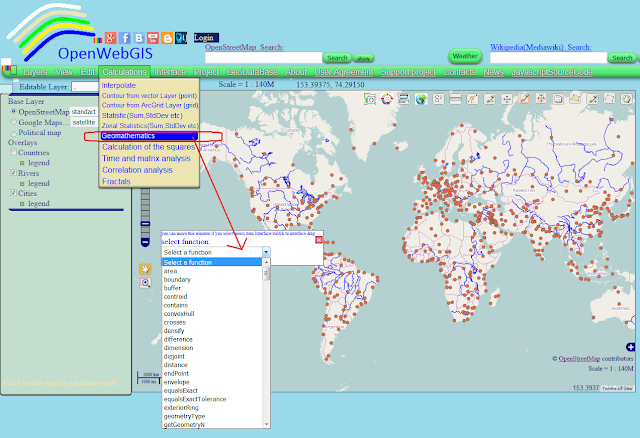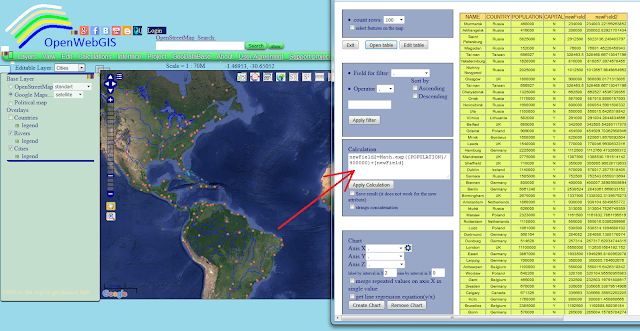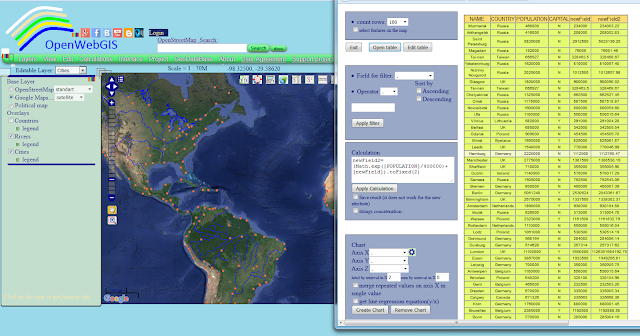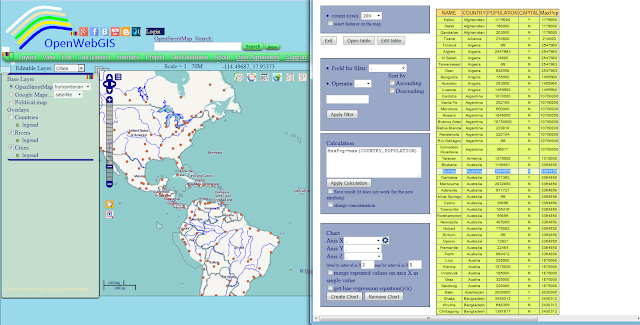## Saturday, 5 December 2015

### Mathematical operations in OpenWebGIS (on the attributes of map layers)

In the description of geographic information system OpenWebGIS there is often a phrase:
"In OpenWebGIS you can use the extensive mathematical functionality to calculate and analyze your data."
It is written because OpenWebGIS has a large set of such functions, among them there are geomathematics functions and mathematical operations on the attributes of map layers. OpenWebGIS (only in the online version) has a large set of geomathematics functions (functions as WPS, provided by Geoserver, and work with them is automated by OpenWebGIS). This function is available through menu item "Calculation->Geomathematics". See Figure 1.p { margin-bottom: 0.25cm; line-height: 120%; } Figure 1- List of geomathematics functions in OpenWebGIS
About each of these functions it is possible to write a separate article, starting with the scientific description of its algorithm and finishing by the method with the help of which the result is added to the map. But in this article we will dwell on the description of mathematical operations on the attributes of map layers. To make mathematical operations on the attributes of the layer, you need to select the name of the layer in the list of "Editable Layer", and then the menu item "Edit-> Open attribute table". Then a window will open for working with attribute information and attribute table. This window is shown in Figure 2.p { margin-bottom: 0.25cm; line-height: 120%; } Figure 2 - Attribute table of the Layer

Pay attention to the block called "Calculation". In this block in text area, you can write mathematical functions, operations to fulfil mathematical calculations on the existing values of the attributes of a layer or create a new attribute. These functions and operations must be recorded according to certain rules.
Names of all attributes must be put in square brackets. Name of the new attribute must be without any brackets. You can apply simple arithmetic operations. For example: calc=[LATITUDE]/[LONGITUDE]*100+[LATITUDE]. In this example 'calc' may be a new attribute (field), then it is added to the list of layer attributes, or it already exists and then all the previous attribute values are replaced with new ones.
Also use the functions: Math.sin([newField]), Math.abs([newField]), Math.cos([newField]), Math.random(), Math.exp(([newField]) - natural exponential, Math.sqrt([newField]), Math.log([newField]) - natural logarithm, Math.pow([newField]),x) - exponentiation x. Example:
newField=(Math.cos([SomeField1])/Math.abs([SomeField2]))+Math.sqrt(16)-Math.sqrt([SomeField3]). To round numbers, you can use the notation: newField=[newField].toFixed (x), where x is the desired number of decimal places. You can also use special formulas: mean, min, max, count. To find the average value for all rows in a column (attribute) SomeField you need to write newField = mean(SomeField). The square brackets in the title of the column are not needed. To find the average value in the column SomeField1 for each unique column values SomeField2, you need to write newField = mean(SomeField2, SomeField1). Similarly newField=min(SomeField2,SomeField1) and newField= count(SomeField2,SomeField1). This is useful for example to calculate the minimum or maximum, quantity of some value within each year, if you have a layer containing information about many years.
If activate "strings concatenation" checkbox, then resulted attribute will be strings concatenation. You must use apostrophe in both side of the string. Example: calc=[LATITUDE]+'text', then if [LATITUDE] = 24.5 -> calc=24.5text; but if string is a number you may not use an apostrophe. Example: calc=24.5+3 then calc = 24.53 - remember that it concerns the case where the option is active "strings concatenation".
All attribute names in the formula must be fully equivalent to their real names, including taking into account the upper and lowercase letters.
Let's look at real examples of mathematical operations on the attributes of the layer. For easy understanding by users as an example take a layer "Cities", which is added by default to the map of OpenWebGIS. We calculate the value equal to half the number of each city population. To do this in the Calculation field you must write the formula as it is shown in Figure 3 and then click on the button "Apply Calculation".p { margin-bottom: 0.25cm; line-height: 120%; } Figure 3 - Example of a mathematical calculation on the layer attributes

We can make the calculation more difficult, apply natural exponential function (Math.exp), as shown in Figure 4 and then round numbers, as shown in Figure 5.p { margin-bottom: 0.25cm; line-height: 120%; } Figure 4 - Using natural exponential functionp { margin-bottom: 0.25cm; line-height: 120%; } Figure 5 - Round result of calculation

And now let's define for each country, the city in which there is the maximum number of population. For this purpose it is necessary to apply a simple formula MaxPop=max(COUNTRY,POPULATION). The result is shown in Figure 6.p { margin-bottom: 0.25cm; line-height: 120%; } Figure 6 - The mathematical definition of the city with the highest number of inhabitants for each country
In the case of the calculation without aggregate functions (min, max, mean, count) JavaScript code is as shown below. Full function code "ApplyCalc()" you can see at GitHub in the file table.htm here or in the file tableOWG.js here
```
var editLayer=window.opener.edilayerMainLayer;
for (var b=0; b<editLayer.features.length; b++)
{
var strCalc=document.getElementById("textCalc").value;
var strCalcMain=strCalc.split('=');
strCalc=strCalcMain;
var res = strCalc.match(/\[.*?\]/ig);
if(res)
{
var strCalc2=strCalc;
for(it=0;it<res.length;it++)
{var str=res[it].split('[').split(']');str2='\\'+'['+str+'\\'+']';
var regC = new RegExp(str2, 'ig');
var ef=editLayer.features[b];
if (document.getElementById("CheckSaveTextAtt").checked==false)
{
strCalc2=strCalc2.replace(regC,'parseFloat(ef.attributes['+'"'+str+'"'+'])')}
else
{strCalc2=strCalc2.replace(regC,'ef.attributes['+'"'+str+'"'+'].toString()')}
}
} //end if(res)
else{var strCalc2=strCalc;}
strCalcMain=strCalcMain;
editLayer.features[b].attributes[strCalcMain]=eval(strCalc2);
if (document.getElementById("CheckSaveOldAtt").checked==true)
{editLayer.features[b].state = OpenLayers.State.UPDATE;}
var prov=parseInt(-100);
if(typeof(editLayer.features[b].attributes[strCalcMain])=='string')
{ prov=editLayer.features[b].attributes[strCalcMain].indexOf('NaN');}
if(isNaN(editLayer.features[b].attributes[strCalcMain])
&&typeof(editLayer.features[b].attributes[strCalcMain])!='string')
{flagError=1;}
if(prov!==parseInt(-1)&&prov!==parseInt(-100))
{flagError=1;}
}
if(flagError==1)
{alert("ERROR. Names of attributes are not written in
upper or lowercase letters or other error");}
else
{
if (document.getElementById("CheckSaveOldAtt").checked==true)
{window.opener.saveStrategy.layer=editLayer;window.opener.saveStrategy.save();}
1.